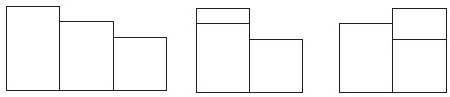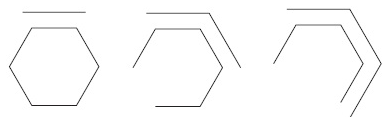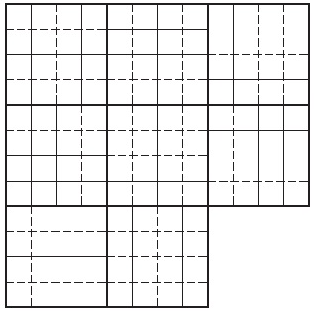# General mental ability - Online Test

Q1. What comes next?Explaination / Solution:

The rectangle starting on the left is moving from left to right one place at a time at each stage.

Q2. Which is the odd one out?
Explaination / Solution:

It has three white and one black on the left and three black and one white on the right. The rest are the opposite way round.

Q3. What comes next?Explaination / Solution:

The inner hexagon is being dismantled one side at a time working anti clockwise, while the outer hexagon is being constructed one side at a time working clockwise.

Q4. Which is the missing tile?Explaination / Solution:

Looking across and down, lines are carried forward from the first two squares to the final square when they appear in the same position twice in the first two squares, however, they then change from complete to broken lines and vice versa.

Q5. What number should replace the question mark?Explaination / Solution:

The number formed at the top is half of the number formed at the bottom i.e. 358 x 2 = 716

Q6. Which is the missing tile?Explaination / Solution:

Each line and column contains one each of the four different lines.

Q7. A candidate attempted 12 questions and secured full marks in all of them. If he obtained 60% in the test and all questions carried equal marks, then what is the number of questions in the test?
Explaination / Solution:
No Explaination.

Q8. A cuboid has six sides of different colours. The red side is opposite to black. The blue side is adjacent to white. The brown side is adjacent to blue. The red side is face down. Which one of the following would be the opposite to brown?
Explaination / Solution:
No Explaination.

Q9. A man fills a basket with eggs in such a way that the number of eggs added on each successive day is the same as the number already present in the basket. This way the basket gets completely filled in 24 days. After how many days the basket was 1 / 4th full?
Explaination / Solution:
No Explaination.

Q10. A person has 4 coins each of different denomination. What is the number of different sums of money the person can form (using one or more coins at a time)?
Explaination / Solution:
No Explaination.